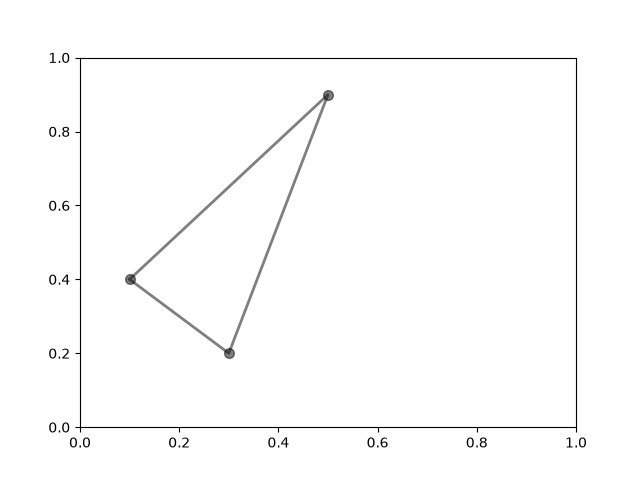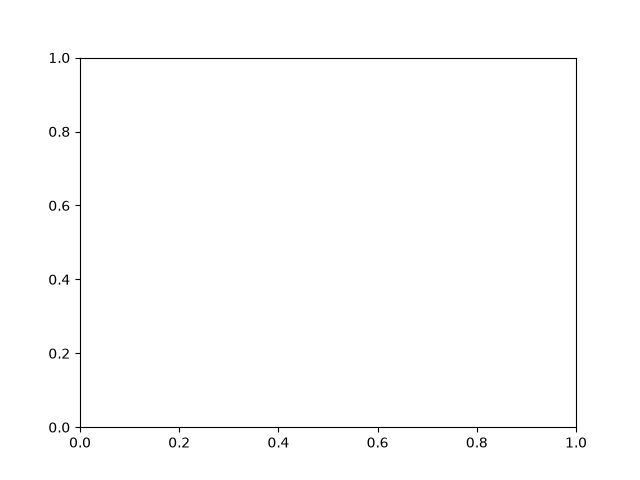# Polygon Selector#

Shows how to create a polygon programmatically or interactively

import matplotlib.pyplot as plt
from matplotlib.widgets import PolygonSelector


To create the polygon programmatically

fig, ax = plt.subplots()
fig.show()

selector = PolygonSelector(ax, lambda *args: None)

selector.verts = [(0.1, 0.4), (0.5, 0.9), (0.3, 0.2)]To create the polygon interactively

fig2, ax2 = plt.subplots()
fig2.show()

selector2 = PolygonSelector(ax2, lambda *args: None)

print("Click on the figure to create a polygon.")
print("Press the 'esc' key to start a new polygon.")
print("Try holding the 'shift' key to move all of the vertices.")
print("Try holding the 'ctrl' key to move a single vertex.")Click on the figure to create a polygon.
Press the 'esc' key to start a new polygon.
Try holding the 'shift' key to move all of the vertices.
Try holding the 'ctrl' key to move a single vertex.


References

The use of the following functions, methods, classes and modules is shown in this example:

Gallery generated by Sphinx-Gallery